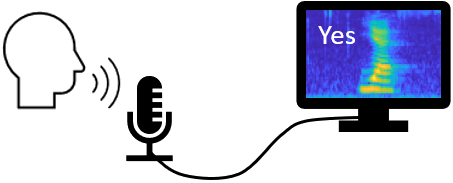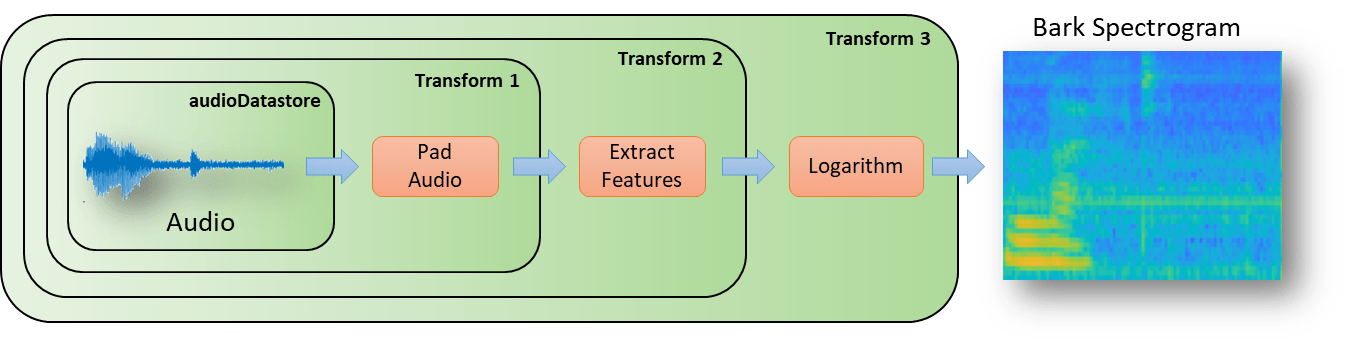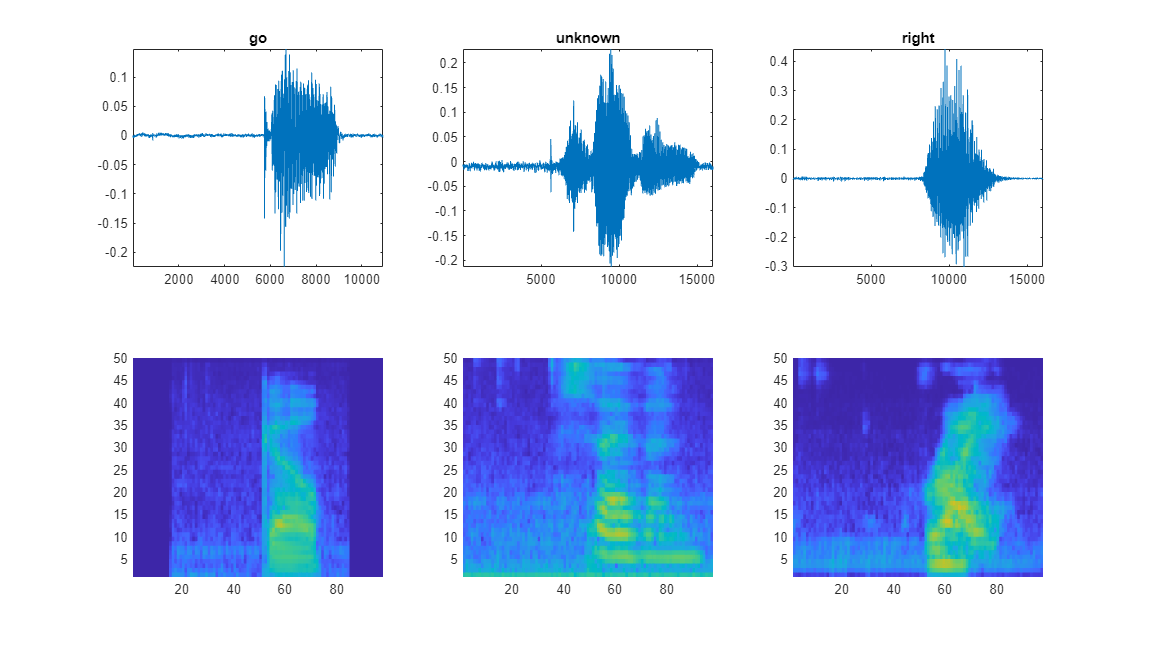# Speech Command Recognition Using Deep Learning

This example shows how to train a deep learning model that detects the presence of speech commands in audio. The example uses the Speech Commands Dataset  to train a convolutional neural network to recognize a given set of commands.

To train a network from scratch, you must first download the data set. If you do not want to download the data set or train the network, then you can load a pretrained network provided with this example and execute the next two sections of the example: Recognize Commands Using Pre-Trained Network and Detect Commands Using Streaming Audio from Microphone.

### Recognize Commands Using Pre-Trained Network

Before going into the training process in detail, use a pre-trained speech recognition network to identify speech commands.

`load("commandNet.mat")`

The network is trained to recognize the following speech commands: yes, no, up, down, left, right, on, off, stop, and go.

Load a short speech signal where a person says stop.

`[x,fs] = audioread("stop_command.flac");`

Listen to the command.

`sound(x,fs)`

The pre-trained network takes auditory-based spectrograms as inputs. You will first convert the speech waveform to an auditory-based spectrogram.

Use the function `helperExtractAuditoryFeature` to compute the auditory spectrogram. You will go through the details of feature extraction later in the example.

`auditorySpect = helperExtractAuditoryFeatures(x,fs);`

Classify the command based on its auditory spectrogram.

`command = classify(trainedNet,auditorySpect)`
```command = categorical stop ```

The network is trained to classify words not belonging to this set as unknown.

You will now classify a word (play) that was not included in the list of command to identify.

First, load the speech signal and listen to it.

```x = audioread("play_command.flac"); sound(x,fs)```

Compute the auditory spectrogram.

`auditorySpect = helperExtractAuditoryFeatures(x,fs);`

Classify the signal.

`command = classify(trainedNet,auditorySpect)`
```command = categorical unknown ```

The network is trained to classify background noise as background.

Create a one-second signal consisting of random noise.

`x = pinknoise(16e3);`

Compute the auditory spectrogram.

`auditorySpect = helperExtractAuditoryFeatures(x,fs);`

Classify the background noise.

`command = classify(trainedNet,auditorySpect)`
```command = categorical background ```

### Detect Commands Using Streaming Audio from Microphone

Test your pre-trained command detection network on streaming audio from your microphone. Try saying one of the commands, for example, yes, no, or stop. Then, try saying one of the unknown words such as Marvin, Sheila, bed, house, cat, bird, or any number from zero to nine.

Specify the classification rate in Hz and create an audio device reader that can read audio from your microphone.

```classificationRate = 20; adr = audioDeviceReader(SampleRate=fs,SamplesPerFrame=floor(fs/classificationRate));```

Initialize a buffer for the audio. Extract the classification labels of the network. Initialize buffers of half a second for the labels and classification probabilities of the streaming audio. Use these buffers to compare the classification results over a longer period of time and by that build 'agreement' over when a command is detected. Specify thresholds for the decision logic.

```audioBuffer = dsp.AsyncBuffer(fs); labels = trainedNet.Layers(end).Classes; YBuffer(1:classificationRate/2) = categorical("background"); probBuffer = zeros([numel(labels),classificationRate/2]); countThreshold = ceil(classificationRate*0.2); probThreshold = 0.7;```

Create a `timescope` object to visualize the audio input from your microphone. Create a `dsp.MatrixViewer` object to visualize the auditory spectrogram used to make predictions.

```wavePlotter = timescope( ... SampleRate=fs, ... Title="...", ... TimeSpanSource="property", ... TimeSpan=1, ... YLimits=[-1,1], ... Position=[600,640,800,340], ... TimeAxisLabels="none", ... AxesScaling="manual"); show(wavePlotter) specPlotter = dsp.MatrixViewer( ... XDataMode="Custom", ... AxisOrigin="Lower left corner", ... Position=[600,220,800,380], ... ShowGrid=false, ... Title="...", ... XLabel="Time (s)", ... YLabel="Bark (bin)"); show(specPlotter)```

Perform live speech command recognition using audio input from your microphone. To run the loop indefinitely, set `timeLimit` to `Inf`. To stop the live detection, close the timescope and `dsp.MatrixViewer` figures.

```% Initialize variables for plotting currentTime = 0; colorLimits = [-1,1]; timeLimit = 10; tic while toc<timeLimit && isVisible(wavePlotter) && isVisible(specPlotter) % Extract audio samples from the audio device and add the samples to % the buffer. x = adr(); write(audioBuffer,x); y = read(audioBuffer,fs,fs-adr.SamplesPerFrame); spec = helperExtractAuditoryFeatures(y,fs); % Classify the current spectrogram, save the label to the label buffer, % and save the predicted probabilities to the probability buffer. [YPredicted,probs] = classify(trainedNet,spec,ExecutionEnvironment="cpu"); YBuffer = [YBuffer(2:end),YPredicted]; probBuffer = [probBuffer(:,2:end),probs(:)]; % Plot the current waveform and spectrogram. wavePlotter(y(end-adr.SamplesPerFrame+1:end)) specPlotter(spec') % Now do the actual command detection by performing a thresholding operation. % Declare a detection and display it in the figure if the following hold: % 1) The most common label is not background. % 2) At last countThreshold of the latest frame labels agree. % 3) The maximum probability of the predicted label is at least probThreshold. % Otherwise, do not declare a detection. [YMode,count] = mode(YBuffer); maxProb = max(probBuffer(labels == YMode,:)); if YMode == "background" || count < countThreshold || maxProb < probThreshold wavePlotter.Title = "..."; specPlotter.Title = "..."; else wavePlotter.Title = string(YMode); specPlotter.Title = string(YMode); end % Update variables for plotting currentTime = currentTime + adr.SamplesPerFrame/fs; colorLimits = [min([colorLimits(1),min(spec,[],"all")]),max([colorLimits(2),max(spec,[],"all")])]; specPlotter.CustomXData = [currentTime-1,currentTime]; specPlotter.ColorLimits = colorLimits; end release(wavePlotter)````release(specPlotter)`### Load Speech Commands Data Set

```downloadFolder = matlab.internal.examples.downloadSupportFile("audio","google_speech.zip"); dataFolder = tempdir; unzip(downloadFolder,dataFolder) dataset = fullfile(dataFolder,"google_speech");```

### Create Training Datastore

Create an `audioDatastore` that points to the training data set.

```ads = audioDatastore(fullfile(dataset,"train"), ... IncludeSubfolders=true, ... FileExtensions=".wav", ... LabelSource="foldernames")```
```ads = audioDatastore with properties: Files: { ' ...\AppData\Local\Temp\google_speech\train\bed\00176480_nohash_0.wav'; ' ...\AppData\Local\Temp\google_speech\train\bed\004ae714_nohash_0.wav'; ' ...\AppData\Local\Temp\google_speech\train\bed\004ae714_nohash_1.wav' ... and 51085 more } Folders: { 'C:\Users\bhemmat\AppData\Local\Temp\google_speech\train' } Labels: [bed; bed; bed ... and 51085 more categorical] AlternateFileSystemRoots: {} OutputDataType: 'double' SupportedOutputFormats: ["wav" "flac" "ogg" "mp4" "m4a"] DefaultOutputFormat: "wav" ```

### Choose Words to Recognize

Specify the words that you want your model to recognize as commands. Label all words that are not commands as `unknown`. Labeling words that are not commands as `unknown` creates a group of words that approximates the distribution of all words other than the commands. The network uses this group to learn the difference between commands and all other words.

To reduce the class imbalance between the known and unknown words and speed up processing, only include a fraction of the unknown words in the training set.

Use `subset` to create a datastore that contains only the commands and the subset of unknown words. Count the number of examples belonging to each category.

```commands = categorical(["yes","no","up","down","left","right","on","off","stop","go"]); isCommand = ismember(ads.Labels,commands); isUnknown = ~isCommand; includeFraction = 0.2; mask = rand(numel(ads.Labels),1) < includeFraction; isUnknown = isUnknown & mask; ads.Labels(isUnknown) = categorical("unknown"); adsTrain = subset(ads,isCommand|isUnknown); countEachLabel(adsTrain)```
```ans=11×2 table Label Count _______ _____ down 1842 go 1861 left 1839 no 1853 off 1839 on 1864 right 1852 stop 1885 unknown 6490 up 1843 yes 1860 ```

### Create Validation Datastore

Create an `audioDatastore` that points to the validation data set. Follow the same steps used to create the training datastore.

```ads = audioDatastore(fullfile(dataset,"validation"), ... IncludeSubfolders=true, ... FileExtensions=".wav", ... LabelSource="foldernames")```
```ads = audioDatastore with properties: Files: { ' ...\AppData\Local\Temp\google_speech\validation\bed\026290a7_nohash_0.wav'; ' ...\AppData\Local\Temp\google_speech\validation\bed\060cd039_nohash_0.wav'; ' ...\AppData\Local\Temp\google_speech\validation\bed\060cd039_nohash_1.wav' ... and 6795 more } Folders: { 'C:\Users\bhemmat\AppData\Local\Temp\google_speech\validation' } Labels: [bed; bed; bed ... and 6795 more categorical] AlternateFileSystemRoots: {} OutputDataType: 'double' SupportedOutputFormats: ["wav" "flac" "ogg" "mp4" "m4a"] DefaultOutputFormat: "wav" ```
```isCommand = ismember(ads.Labels,commands); isUnknown = ~isCommand; includeFraction = 0.2; mask = rand(numel(ads.Labels),1) < includeFraction; isUnknown = isUnknown & mask; ads.Labels(isUnknown) = categorical("unknown"); adsValidation = subset(ads,isCommand|isUnknown); countEachLabel(adsValidation)```
```ans=11×2 table Label Count _______ _____ down 264 go 260 left 247 no 270 off 256 on 257 right 256 stop 246 unknown 828 up 260 yes 261 ```

To train the network with the entire dataset and achieve the highest possible accuracy, set `speedupExample` to `false`. To run this example quickly, set `speedupExample` to `true`.

```speedupExample =false; if speedupExample numUniqueLabels = numel(unique(adsTrain.Labels)); % Reduce the dataset by a factor of 20 adsTrain = splitEachLabel(adsTrain,round(numel(adsTrain.Files)/numUniqueLabels/20)); adsValidation = splitEachLabel(adsValidation,round(numel(adsValidation.Files)/numUniqueLabels/20)); end```

### Compute Auditory Spectrograms

To prepare the data for efficient training of a convolutional neural network, convert the speech waveforms to auditory-based spectrograms.

Define the parameters of the feature extraction. `segmentDuration` is the duration of each speech clip (in seconds). `frameDuration` is the duration of each frame for spectrum calculation. `hopDuration` is the time step between each spectrum. `numBands` is the number of filters in the auditory spectrogram.

Create an `audioFeatureExtractor` object to perform the feature extraction.

```fs = 16e3; % Known sample rate of the data set. segmentDuration = 1; frameDuration = 0.025; hopDuration = 0.010; segmentSamples = round(segmentDuration*fs); frameSamples = round(frameDuration*fs); hopSamples = round(hopDuration*fs); overlapSamples = frameSamples - hopSamples; FFTLength = 512; numBands = 50; afe = audioFeatureExtractor( ... SampleRate=fs, ... FFTLength=FFTLength, ... Window=hann(frameSamples,"periodic"), ... OverlapLength=overlapSamples, ... barkSpectrum=true); setExtractorParameters(afe,"barkSpectrum",NumBands=numBands,WindowNormalization=false);```

Read a file from the dataset. Training a convolutional neural network requires input to be a consistent size. Some files in the data set are less than 1 second long. Apply zero-padding to the front and back of the audio signal so that it is of length `segmentSamples`.

```x = read(adsTrain); numSamples = size(x,1); numToPadFront = floor((segmentSamples - numSamples)/2); numToPadBack = ceil((segmentSamples - numSamples)/2); xPadded = [zeros(numToPadFront,1,"like",x);x;zeros(numToPadBack,1,"like",x)];```

To extract audio features, call `extract`. The output is a Bark spectrum with time across rows.

```features = extract(afe,xPadded); [numHops,numFeatures] = size(features)```
```numHops = 98 ```
```numFeatures = 50 ```

In this example, you post-process the auditory spectrogram by applying a logarithm. Taking a log of small numbers can lead to roundoff error.

To speed up processing, you can distribute the feature extraction across multiple workers using `parfor`.

First, determine the number of partitions for the dataset. If you do not have Parallel Computing Toolbox™, use a single partition.

```if ~isempty(ver("parallel")) && ~speedupExample pool = gcp; numPar = numpartitions(adsTrain,pool); else numPar = 1; end```

For each partition, read from the datastore, zero-pad the signal, and then extract the features.

```parfor ii = 1:numPar subds = partition(adsTrain,numPar,ii); XTrain = zeros(numHops,numBands,1,numel(subds.Files)); for idx = 1:numel(subds.Files) x = read(subds); xPadded = [zeros(floor((segmentSamples-size(x,1))/2),1);x;zeros(ceil((segmentSamples-size(x,1))/2),1)]; XTrain(:,:,:,idx) = extract(afe,xPadded); end XTrainC{ii} = XTrain; end```

Convert the output to a 4-dimensional array with auditory spectrograms along the fourth dimension.

```XTrain = cat(4,XTrainC{:}); [numHops,numBands,numChannels,numSpec] = size(XTrain)```
```numHops = 98 ```
```numBands = 50 ```
```numChannels = 1 ```
```numSpec = 25028 ```

Scale the features by the window power and then take the log. To obtain data with a smoother distribution, take the logarithm of the spectrograms using a small offset.

```epsil = 1e-6; XTrain = log10(XTrain + epsil);```

Perform the feature extraction steps described above to the validation set.

```if ~isempty(ver("parallel")) pool = gcp; numPar = numpartitions(adsValidation,pool); else numPar = 1; end parfor ii = 1:numPar subds = partition(adsValidation,numPar,ii); XValidation = zeros(numHops,numBands,1,numel(subds.Files)); for idx = 1:numel(subds.Files) x = read(subds); xPadded = [zeros(floor((segmentSamples-size(x,1))/2),1);x;zeros(ceil((segmentSamples-size(x,1))/2),1)]; XValidation(:,:,:,idx) = extract(afe,xPadded); end XValidationC{ii} = XValidation; end XValidation = cat(4,XValidationC{:}); XValidation = log10(XValidation + epsil);```

Isolate the train and validation labels. Remove empty categories.

```TTrain = removecats(adsTrain.Labels); TValidation = removecats(adsValidation.Labels);```

### Visualize Data

Plot the waveforms and auditory spectrograms of a few training samples. Play the corresponding audio clips.

```specMin = min(XTrain,[],"all"); specMax = max(XTrain,[],"all"); idx = randperm(numel(adsTrain.Files),3); figure(Units="normalized",Position=[0.2 0.2 0.6 0.6]); for ii = 1:3 [x,fs] = audioread(adsTrain.Files{idx(ii)}); subplot(2,3,ii) plot(x) axis tight title(string(adsTrain.Labels(idx(ii)))) subplot(2,3,ii+3) spect = XTrain(:,:,1,idx(ii))'; pcolor(spect) caxis([specMin specMax]) shading flat sound(x,fs) pause(2) end```The network must be able not only to recognize different spoken words but also to detect if the input contains silence or background noise.

Use the audio files in the background folder to create samples of one-second clips of background noise. Create an equal number of background clips from each background noise file. You can also create your own recordings of background noise and add them to the background folder. Before calculating the spectrograms, the function rescales each audio clip with a factor sampled from a log-uniform distribution in the range given by `volumeRange`.

`adsBkg = audioDatastore(fullfile(dataset,"background"))`
```adsBkg = audioDatastore with properties: Files: { ' ...\AppData\Local\Temp\google_speech\background\doing_the_dishes.wav'; ' ...\bhemmat\AppData\Local\Temp\google_speech\background\dude_miaowing.wav'; ' ...\bhemmat\AppData\Local\Temp\google_speech\background\exercise_bike.wav' ... and 3 more } Folders: { 'C:\Users\bhemmat\AppData\Local\Temp\google_speech\background' } AlternateFileSystemRoots: {} OutputDataType: 'double' Labels: {} SupportedOutputFormats: ["wav" "flac" "ogg" "mp4" "m4a"] DefaultOutputFormat: "wav" ```
```numBkgClips = 4000; if speedupExample numBkgClips = numBkgClips/20; end volumeRange = log10([1e-4,1]); numBkgFiles = numel(adsBkg.Files); numClipsPerFile = histcounts(1:numBkgClips,linspace(1,numBkgClips,numBkgFiles+1)); Xbkg = zeros(size(XTrain,1),size(XTrain,2),1,numBkgClips,"single"); bkgAll = readall(adsBkg); ind = 1; for count = 1:numBkgFiles bkg = bkgAll{count}; idxStart = randi(numel(bkg)-fs,numClipsPerFile(count),1); idxEnd = idxStart+fs-1; gain = 10.^((volumeRange(2)-volumeRange(1))*rand(numClipsPerFile(count),1) + volumeRange(1)); for j = 1:numClipsPerFile(count) x = bkg(idxStart(j):idxEnd(j))*gain(j); x = max(min(x,1),-1); Xbkg(:,:,:,ind) = extract(afe,x); if mod(ind,1000)==0 progress = "Processed " + string(ind) + " background clips out of " + string(numBkgClips) end ind = ind + 1; end end```
```progress = "Processed 1000 background clips out of 4000" ```
```progress = "Processed 2000 background clips out of 4000" ```
```progress = "Processed 3000 background clips out of 4000" ```
```progress = "Processed 4000 background clips out of 4000" ```
`Xbkg = log10(Xbkg + epsil);`

Split the spectrograms of background noise between the training, validation, and test sets. Because the background noise folder contains only about five and a half minutes of background noise, the background samples in the different data sets are highly correlated. To increase the variation in the background noise, you can create your own background files and add them to the folder. To increase the robustness of the network to noise, you can also try mixing background noise into the speech files.

```numTrainBkg = floor(0.85*numBkgClips); numValidationBkg = floor(0.15*numBkgClips); XTrain(:,:,:,end+1:end+numTrainBkg) = Xbkg(:,:,:,1:numTrainBkg); TTrain(end+1:end+numTrainBkg) = "background"; XValidation(:,:,:,end+1:end+numValidationBkg) = Xbkg(:,:,:,numTrainBkg+1:end); TValidation(end+1:end+numValidationBkg) = "background";```

Plot the distribution of the different class labels in the training and validation sets.

```figure(Units="normalized",Position=[0.2 0.2 0.5 0.5]) tiledlayout(2,1) nexttile histogram(TTrain) title("Training Label Distribution") nexttile histogram(TValidation) title("Validation Label Distribution")```### Define Neural Network Architecture

Create a simple network architecture as an array of layers. Use convolutional and batch normalization layers, and downsample the feature maps "spatially" (that is, in time and frequency) using max pooling layers. Add a final max pooling layer that pools the input feature map globally over time. This enforces (approximate) time-translation invariance in the input spectrograms, allowing the network to perform the same classification independent of the exact position of the speech in time. Global pooling also significantly reduces the number of parameters in the final fully connected layer. To reduce the possibility of the network memorizing specific features of the training data, add a small amount of dropout to the input to the last fully connected layer.

The network is small, as it has only five convolutional layers with few filters. `numF` controls the number of filters in the convolutional layers. To increase the accuracy of the network, try increasing the network depth by adding identical blocks of convolutional, batch normalization, and ReLU layers. You can also try increasing the number of convolutional filters by increasing `numF`.

To give each class equal total weight in the loss, use class weights that are inversely proportional to the number of training examples in each class. When using the Adam optimizer to train the network, the training algorithm is independent of the overall normalization of the class weights.

```classes = categories(TTrain); classWeights = 1./countcats(TTrain); classWeights = classWeights'/mean(classWeights); numClasses = numel(categories(TTrain)); timePoolSize = ceil(numHops/8); dropoutProb = 0.2; numF = 12; layers = [ imageInputLayer([numHops numBands]) convolution2dLayer(3,numF,Padding="same") batchNormalizationLayer reluLayer maxPooling2dLayer(3,Stride=2,Padding="same") convolution2dLayer(3,2*numF,Padding="same") batchNormalizationLayer reluLayer maxPooling2dLayer(3,Stride=2,Padding="same") convolution2dLayer(3,4*numF,Padding="same") batchNormalizationLayer reluLayer maxPooling2dLayer(3,Stride=2,Padding="same") convolution2dLayer(3,4*numF,Padding="same") batchNormalizationLayer reluLayer convolution2dLayer(3,4*numF,Padding="same") batchNormalizationLayer reluLayer maxPooling2dLayer([timePoolSize,1]) dropoutLayer(dropoutProb) fullyConnectedLayer(numClasses) softmaxLayer classificationLayer(Classes=classes,ClassWeights=classWeights)];```

### Train Network

Specify the training options. Use the Adam optimizer with a mini-batch size of 128. Train for 25 epochs and reduce the learning rate by a factor of 10 after 20 epochs.

```miniBatchSize = 128; validationFrequency = floor(numel(TTrain)/miniBatchSize); options = trainingOptions("adam", ... InitialLearnRate=3e-4, ... MaxEpochs=25, ... MiniBatchSize=miniBatchSize, ... Shuffle="every-epoch", ... Plots="training-progress", ... Verbose=false, ... ValidationData={XValidation,TValidation}, ... ValidationFrequency=validationFrequency, ... LearnRateSchedule="piecewise", ... LearnRateDropFactor=0.1, ... LearnRateDropPeriod=20);```

Train the network. If you do not have a GPU, then training the network can take time.

`trainedNet = trainNetwork(XTrain,TTrain,layers,options);`### Evaluate Trained Network

Calculate the final accuracy of the network on the training set (without data augmentation) and validation set. The network is very accurate on this data set. However, the training, validation, and test data all have similar distributions that do not necessarily reflect real-world environments. This limitation particularly applies to the `unknown` category, which contains utterances of only a small number of words.

```if speedupExample load("commandNet.mat","trainedNet"); end YValPred = classify(trainedNet,XValidation); validationError = mean(YValPred ~= TValidation); YTrainPred = classify(trainedNet,XTrain); trainError = mean(YTrainPred ~= TTrain); disp("Training error: " + trainError*100 + "%")```
```Training error: 1.5794% ```
`disp("Validation error: " + validationError*100 + "%")`
```Validation error: 4.6692% ```

Plot the confusion matrix. Display the precision and recall for each class by using column and row summaries. Sort the classes of the confusion matrix. The largest confusion is between unknown words and commands, up and off, down and no, and go and no.

```figure(Units="normalized",Position=[0.2 0.2 0.5 0.5]); cm = confusionchart(TValidation,YValPred, ... Title="Confusion Matrix for Validation Data", ... ColumnSummary="column-normalized",RowSummary="row-normalized"); sortClasses(cm,[commands,"unknown","background"])```When working on applications with constrained hardware resources such as mobile applications, consider the limitations on available memory and computational resources. Compute the total size of the network in kilobytes and test its prediction speed when using a CPU. The prediction time is the time for classifying a single input image. If you input multiple images to the network, these can be classified simultaneously, leading to shorter prediction times per image. When classifying streaming audio, however, the single-image prediction time is the most relevant.

```info = whos("trainedNet"); disp("Network size: " + info.bytes/1024 + " kB")```
```Network size: 292.2139 kB ```
```time = zeros(100,1); for ii = 1:100 x = randn([numHops,numBands]); tic [YPredicted,probs] = classify(trainedNet,x,ExecutionEnvironment="cpu"); time(ii) = toc; end disp("Single-image prediction time on CPU: " + mean(time(11:end))*1000 + " ms")```
```Single-image prediction time on CPU: 2.4838 ms ```